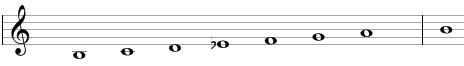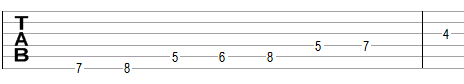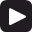# Altered scale

The altered scale is a secondary heptatonic scale that can be built using the following steps between notes: ½, 1, ½, 1, 1, 1, 1.

One example of the altered scale is B, C, D, Eb, F, G, A. This example is shown below in traditional notation and in guitar tablature notation.The altered scale is essentially the Locrian scale B, C, D, E, F, G, A, but with the flat fourth Eb.

Click Play to hear the altered scale.## Modes of the altered scale

The following are modes of the altered scale.

1. Altered, or Locrian with a flat fourth, with steps ½, 1, ½, 1, 1, 1, 1 (B, C, D, Eb, F, G, A in the example above).
2. Melodic ascending minor, with steps 1, ½, 1, 1, 1, 1, ½ (C, D, Eb, F, G, A, B).
3. Phrygian with a raised sixth, with steps ½, 1, 1, 1, 1, ½, 1 (D, Eb, F, G, A, B, C).
4. Lydian with a raised fifth, with steps 1, 1, 1, 1, ½, 1, ½ (Eb, F, G, A, B, C, D).
5. Lydian-Mixolydian, also known as "acoustic", with steps 1, 1, 1, ½, 1, ½, 1 (F, G, A, B, C, D, Eb).
6. Major-minor (minor with a raised or major third), with steps 1, 1, ½, 1, ½, 1, 1 (G, A, B, C, D, Eb, F).
7. Locrian with a raised second, with steps 1, ½, 1, ½, 1, 1, 1 (A, B, C, D, Eb, F, G).

## Three-note chords on the altered scale

The following are common triads built on the notes of the altered scale.

• On the root of the scale (on the tonic): diminished chord (e.g., Bdim composed of B, D, F) or augmented chord (e.g., Baug = B, Eb, G).
• On the second note (on the supertonic): minor chord (e.g., Cm = C, Eb, G) or suspended chord (Csus4 = C, F, G and Csus2 = C, D, G).
• On the third note (on the mediant): minor chord (e.g., Dm = D, F, A) or suspended chord (Dsus4 = D, G, A).
• On the fourth note (on the subdominant): augmented chord (e.g., Ebaug = Eb, G, B).
• On the fifth note (on the dominant): major chord (e.g., F = F, A, C) or suspended chord (Fsus2 = F, G, C).
• On the sixth note (on the submediant): major chord on (e.g., G = G, B, D), augmented chord (Gaug = G, B, Eb) or suspended chords (Gsus4 = G, C, D and Gsus2 = G, A, D).
• On the seventh note (on the leading tone): diminished chord (e.g. Adim = A, C, Eb).

## Four-note chords on the altered scale

The following are seventh chords built on the notes of the altered scale.

• On the first note: half-diminished seventh chord (minor seventh chord with a flat fifth, e.g. Bm7b5 composed of B, D, F, A) or an augmented seventh chord (dominant seventh chord with a raised fifth, B7#5 = B, Eb, G, A).
• On the second note: minor-major seventh chord (Cmmaj7 = C, Eb, G, B).
• On the third note: minor seventh chord (Dm7 = D, F, A, C).
• On the fourth note: augmented major seventh chord (major seventh chord with a raised fifth, Ebmaj7#5 = Eb, G, B, D.
• On the fifth note: dominant seventh chord (F7 = F, A, C, Eb).
• On the sixth note: dominant seventh (G7 = G, B, D, F) or augmented seventh chord (G7#5 = G, B, Eb, F).
• On the seventh note: half-diminished seventh chord (Am7b5 = A, C, Eb, G).

## Intervals on the altered scale

The altered scale is composed of the following intervals.

• A minor second, e.g., the interval between B and C is equal to one semitone.
• A minor third, e.g., the interval between B and D is equal to three semitones.
• A diminished fourth, e.g., the interval between B and Eb is equal to four semitones.
• A diminished fifth, e.g., the interval between B and F is equal to six semitones.
• A minor sixth, e.g., the interval between B and G is equal to eight semitones.
• A minor seventh, e.g., the interval between B and A is equal to ten semitones.

Scale, Scale (index)

### Filtered HTML

• Freelinking helps you easily create HTML links. Links take the form of [[indicator:target|Title]]. By default (no indicator): Click to view a local node.